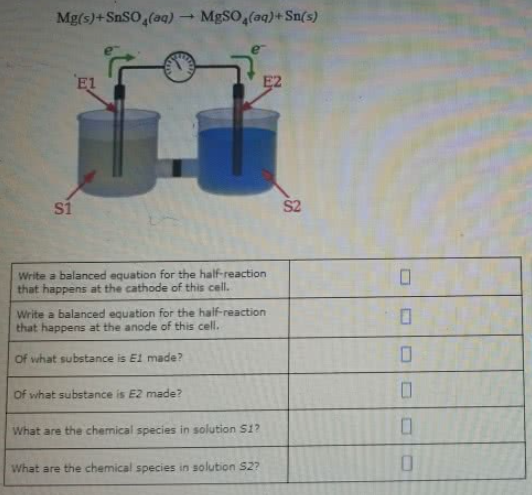# Problem: Suppose the galvanic cell sketched below is powered by the following reaction Mg(s)+SnSO4(aq) → MgSO4(aq) + Sn(s) Write a balanced equation for the half-reaction that happens at the cathode of this cell. Write a balanced equation for the half-reaction that happens at the anode of this cell. Of what substance is E1 made? of what substance is E2 made?What are the chemical species in solution S1? What are the chemical species in solution S2?

###### FREE Expert Solution

 Anode is in the negative side (left side of the diagram)

▪ Anode → oxidation

oxidation half-reaction happens in the anode solution

▪ losing electrons → becoming more positive → anode dissolves away

 Cathode is in the positive side (right side of the diagram)

▪ Cathode → reduction

reduction half-reaction happens in the anode solution

▪ gaining electrons → becoming more negative → solids is deposited in the surface

85% (497 ratings)###### Problem DetailsSuppose the galvanic cell sketched below is powered by the following reaction

Mg(s)+SnSO4(aq) → MgSO4(aq) + Sn(s)

Write a balanced equation for the half-reaction that happens at the cathode of this cell.

Write a balanced equation for the half-reaction that happens at the anode of this cell.

Of what substance is E1 made?

of what substance is E2 made?

What are the chemical species in solution S1?

What are the chemical species in solution S2?